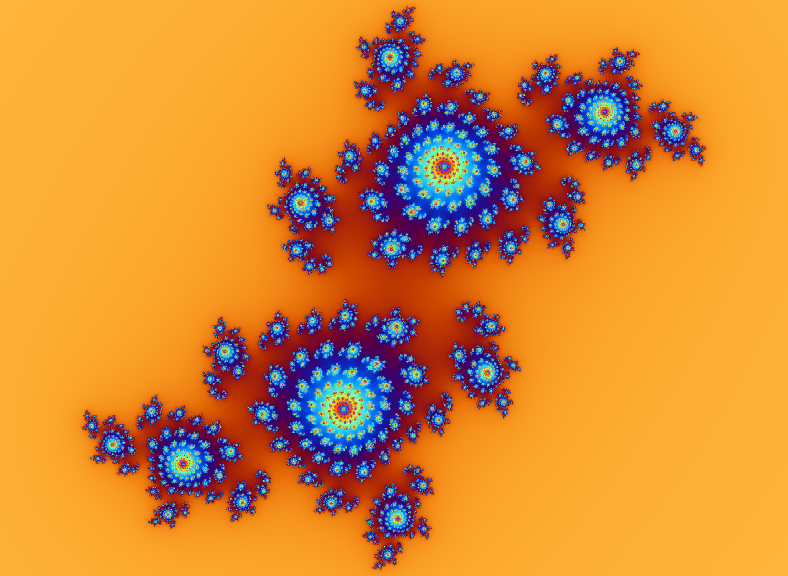# mathr / blog / #

## GPU Julia SetsI found a nice image illustrating the connection between Julia sets and the Mandelbrot set. So I set about implementing Julia set rendering on the GPU. Here's the fragment shader I came up with:

uniform sampler2D coords;  // z-plane iterates
uniform vec2 c;   // complex c for the iteration: z -> z^2 + c
uniform float r;  // escape radius
uniform float n;  // iteration count
void main(void) {
vec2 p = gl_TexCoord.st;
vec4 q = texture2D(coords, p);
vec4 o;
if (q.a < 0.5) { // use alpha to flag escapees
vec2 z = q.rg;
vec2 zz = vec2(z.x * z.x - z.y * z.y, 2.0 * z.x * z.y) + c;
float d = sqrt(dot(zz,zz));
if (d <= r) { // still not escaped
o = vec4(zz, 0.0, 0.0);
} else { // colour escapee according to escape velocity
float v = 0.025 * (n - log2(log2(d)/log2(r)));
o = vec4(
cos(sqrt(5.0) * v      ) * 0.5 + 0.5,
cos(sqrt(6.0) * v + 1.0) * 0.5 + 0.5,
cos(sqrt(7.0) * v + 2.0) * 0.5 + 0.5,
1.0
);
}
} else { // pass through unmodified (already escaped and coloured)
o = q;
}
gl_FragData = o;
}

It's a little messy but it does the trick. To use it, initialize a texture with the starting complex coordinates (x,y,0,0) at each point, then the usual ping pong feedback loop. I added some simple oversampling with another shader to do a simple box blur, which I also reused to detect completion of rendering (if say 25 iterations pass without any new escapees, then assume it's as done as it's going to get).

As for speed, it depends on the closeness of the parameter point to the border of the Mandelbrot set or something similar - a 256x256 grid of 32x32 pixel Julia sets took about 75 minutes to render. Here's a 64x64 grid of 64x64 pixel Julia sets, along with some more GPU-calculated Julia set images:

The whole mostly uncommented and hacky source is in Git at maximus/gpujulia at gitorious.org gpujulia at code.mathr.co.uk.